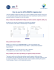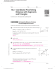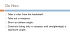# The Midpoint Formula

## Transcription

The Midpoint Formula
```The Midpoint Formula
Name
To find the midpoint between two ordered
pairs, add the x-coordinates and divide by 2,
then add the y-coordinates and divide by 2.
xl+x2
Yt+Yz \
t-t
\2
t2l
-l
of
Find the midpoints for these sets
ordered pairs. Then graph each segment
formed by the two ordered pairs,
checking that the midpoint divides the
segment into two congruent segments.
You will reveal a mathematical symbol
that was introduced in 1525.
Example: Find the midpoint between
t5, -3) and (0, 7).
*=(#,
Segment Endpoints
1.
(10,
7) and
(10, 5)
(-1,
1) and
(1
(-5, -7)
7) and
(0,
(-1
1)
,
(-7,
(1,
-\$ -\$ -? -\$
@
-s
Milliken Publishing CompanY
and
, 5)
(-8, -1)
(10, 7)
and (-5, -7\
1) and
5) and
(-5, -3)
(10, 5)
Midpoint
```

### Solve the problems below to answer the riddle: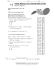### Algebra with shapes How to . . .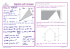### Geometry Worksheet Name: Hodge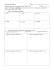### Reteach Sec 1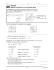### 1-2 Practice A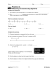### 5.1 Midsegment Theorem and Coordinate Proof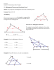### Guidelines TV Lab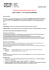### Coordinate Geometry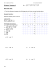### Lesson 30: Special Lines in Triangles M1 Lesson 30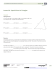### Reteach Sec 1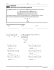### STUDY GUIDE for Unit 1 Test Geometry 1. Z?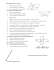### Lesson 12: Dividing Segments Proportionately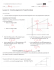### BONSAI WG on Global Impact Assessment (GIA)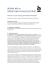### Time: 3 to 3 ½ hours M.M.:90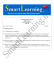### C Geometry Name: 1.4 Worksheet – Constructing Parallel and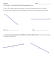### HSM12CC_GM_06_09_CM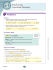### 1.1 Segment Length and Midpoints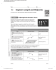### Panel 2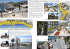### TTCSI Services Talk final proposal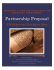### Document 6510483